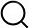# Also in the Article

Model application

Do transportation network companies decrease or increase congestion?

Procedure

After the model was estimated, it was applied to all links to predict the VI:i,t for 2010 and 2016. It was also applied to predict a 2016 counterfactual scenario with no TNCs by setting VTNC:i,2016 and VAvgDur:i,2016 to zero and otherwise applying the model to 2016 data. These predicted PCEs were then used to calculate the travel times using the VDFs (Eq. 1).

The non-PCE volume on each link is calculated as$Vi,t=VSF‐CHAMP:i,t+β2VTNC:i,t$(5)where Vi,t is the traffic volume in units of vehicles instead of PCEs and VSF − CHAMP:i,t is the SF-CHAMP volume. β1 is excluded such that we count the full SF-CHAMP traffic volume, not their estimated effect on speed. The inclusion of β2 (which is less than one) accounts for the partial overlap between the TNC volumes and the background volumes. These volumes were combined with the link lengths to calculate VMT, and combined with travel times to calculate vehicle hours traveled (VHT) and VHD. The average speed is calculated as VMT/VHT. The same volumes were used in combination with observed travel times to calculate observed VHT, VHD, and average speed. In addition, a set of reliability metrics was calculated as described below.

Q&A